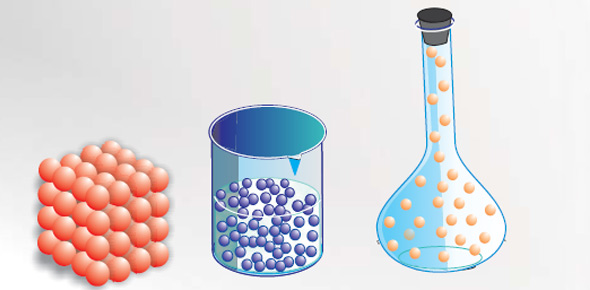# Properties Of Matter Mini Quiz

6 Questions | Total Attempts: 1979SettingsA mini quiz about the properties of matter.

Related Topics
• 1.
1. What is density?
• A.

How much mass is in a given space

• B.

How much matter is in a given space

• 2.
2. What are the three main states of matter?
• A.

Solid, liquid, and gas

• B.

Solid, nitrogen, and liquid

• 3.
3. When sugar is added to water and mixed together it is an example of what kind of change?
• A.

A physical change

• B.

A chemical change

• 4.
4. I put a cup of water in the frezzer and take it out the next day and observe it is now ice. What kind of change is this?
• A.

A chemical change

• B.

A physical change

• 5.
5. When a egg is fried on a pan, the egg represents what?
• A.

A chemical change

• B.

A physical change

• C.

That it is flammable

• 6.
6. When you are identifying the hardness of a mineral you use what?
• A.

A math book

• B.

A ruler and a pencil

• C.

The Mohs scale